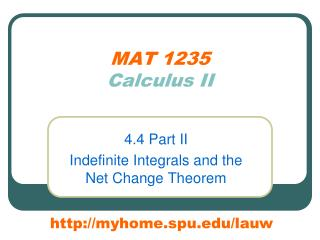# MAT 1235 Calculus II - PowerPoint PPT PresentationDownload PresentationMAT 1235 Calculus II

MAT 1235 Calculus IIDownload Presentation## MAT 1235 Calculus II

- - - - - - - - - - - - - - - - - - - - - - - - - - - E N D - - - - - - - - - - - - - - - - - - - - - - - - - - -
##### Presentation Transcript

1. MAT 1235Calculus II 4.4 Part II Indefinite Integrals and the Net Change Theorem http://myhome.spu.edu/lauw

2. Homework • WebAssign HW 4.4 II

3. Preview • We will discuss the Net Change Theorem • The NCT explains the formulas for computing displacement and distance traveled you have learned in physics.

4. Recall: Fundamental Theorem of Calculus Part 2

5. Recall: Fundamental Theorem of Calculus Part 2

6. Recall: Fundamental Theorem of Calculus Part 2

7. The Net Change Theorem The integral of rate of change is the net change Final value –Initial Value = Net change of from to Rate of change of

8. Example 1: Solution leaking from a tank

9. Example 1 = volume of the solution in the tank at time t = rate of change of the volume

10. Example 2: Linear motion of a particle Reference Frame

11. Example 2:

12. Formulas

13. Recall: Section 2.7 • displacement ≠ distance traveled

14. displacement ≠ distance traveled displacement = distance traveled =

15. Observations distance traveled = displacement =

16. Observations distance traveled = -displacement =

17. Observations: A Unified Formula distance traveled = displacement = distance traveled = -displacement =

18. Formulas

19. Example 3 The velocity function (in meters per second) is given for a particle moving along a line. (a) Find the displacement of the particle. (b) Find the distance traveled. Step 1: Find the interval where v(t)>0 and <0

20. Example 3 Step 2: Use the distance formula

21. Expectations • Please pay attention to the solutions steps. • Do not skip steps. • Do not do multiple things at one step. • It is very helpful to have a diagram.